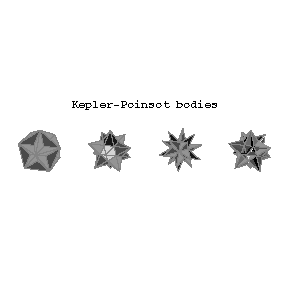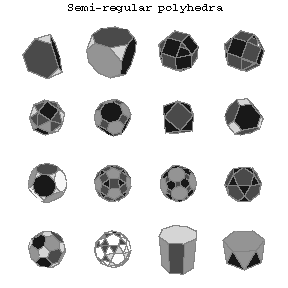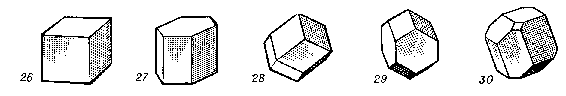# Polyhedron

2010 Mathematics Subject Classification: Primary: 52B [MSN][ZBL]

The union (in a three- or higher-dimensional space) of a finite number of plane polygons such that: 1) each side of any polygon is simultaneously the side of another (and only one), called adjacent to the first (along this side); 2) from any of the polygons forming the polyhedron it is possible to go to any other by passing to an adjacent and from it, in its turn, to an adjacent, etc. These polygons are called the faces, their sides the edges and their vertices the vertices of the polyhedron.

This definition of a polyhedron has different meanings, according to how a polygon is defined. If by a polygon is meant a plane closed polygonal curve (even if self-intersecting), one arrives at the first definition of a polyhedron. Most of this article is constructed on the basis of a second definition of a polyhedron, in which its faces are polygons, understood as parts of planes bounded by polygonal curves. From this point of view a polyhedron is a surface made up of polygonal segments. If this surface does not intersect itself, then it is the full boundary surface of some geometric body, also called a polyhedron; hence a third point of view: a polyhedron as a geometric body where, for these bodies, "holes" bounded by a finite number of plane faces are allowed.

The simplest examples of polyhedra are prisms and pyramids. A polyhedron is called an $n$-gonal pyramid if one of its faces (the base) is an $n$-gon and the other faces are triangles with a common vertex not lying in the plane of the base. A triangular pyramid is also called a tetrahedron. A polyhedron is called an $n$-gonal prism if two of its faces (the bases) are equal $n$-gons (not lying in the same plane), which can be obtained from each other by parallel translation, and the remaining faces are parallelograms, the opposite sides of which are the corresponding sides of the bases. For every polyhedron of genus zero ( "no holes" ) the Euler characteristic (the number of vertices minus the number of edges plus the number of faces) is equal to two; symbolically, $V-E+F=2$ (Euler's theorem). For a polyhedron of genus $p$ one has $V-E+F=2-2p$. A convex polyhedron is the convex hull of a finite number of points, that is, a polyhedron which lies on one side of the plane of each of its faces. Its interior is a convex body. If the surface of a convex body is a polyhedron, then the corresponding polyhedron is convex. The following convex polyhedra are most important.Figure: p073660a

The regular polyhedraFigure: p073660b

The Kepler–Poinsot bodiesFigure: p073660c

The semi-regular polyhedraFigure: p073660d

The convex parallelohedra Regular polyhedra (Platonic solids) are convex polyhedra all faces of which are congruent regular polygons and all polyhedral angles at the vertices are regular and equal (see Fig. a, Regular polyhedra). Isogons and isohedra are convex polygons all polyhedral angles of which are equal (isogons) or all faces are equal (isohedra); moreover, the group of rotations (with reflections) of an isogon (isohedron) around the centre of gravity maps any vertex (face) into any other vertex (face). Each isohedron can be realized so that all its faces are regular polygons. The polyhedra thus obtained are called semi-regular polyhedra (Archimedean solids and their duals) (see Fig. c, Semi-regular polyhedra).

(Convex) parallelohedra (cf. Parallelohedron) are polyhedra which, when considered as bodies, can fill out the whole infinite space by parallel translation so that they do not contain one another and there is no space between them, that is, they form a tiling of the space (see Fig. d, Parallelopipedon).

Based on the first (mentioned at the beginning of this article) definition of a polyhedron it is possible to give also four regular non-convex polyhedra (Kepler–Poinsot bodies). In these polyhedra either the faces intersect each other or the faces themselves are self-intersecting polygons (see Fig. b, Regular polyhedra). In the study of questions related to the areas of surfaces and volumes of polyhedra it is convenient to use just the first definition of a polyhedron.

If in a polyhedron it is possible to orient the faces so that each edge has opposite directions in the two faces which are adjacent along this edge, then the polyhedron is called orientable, otherwise it is called non-orientable (cf. also Orientation). For an orientable polyhedron (even if it is self-intersecting and its faces are self-intersecting polygons) it is possible to introduce the notions of surface area and volume. The surface area of an orientable polyhedron is the sum of the areas of its faces. For the definition of volume one must keep in mind that the union of interior segments of the faces of the polyhedron divide the space into a finite number of connected components, of which one (the exterior) is infinite and the remainder (the interior) are finite. If from an exterior point, relative to a polyhedron, one draws a segment leading to an interior point of an interior component, then the sum of the "coefficients" of the interior components of the faces of the polyhedron which intersect this segment is called the coefficient of this interior component of the polyhedron (it does not depend on the choice of the exterior point); the coefficient is an integer, positive, negative or zero. The sum of the usual volumes of all interior components of the polyhedron, multiplied by their coefficients, is called the volume of the polyhedron.

Polyhedra of dimension $n$ have also been considered. Some of the definitions given here have an $n$-dimensional generalization. In particular, all convex regular polyhedra have been found, for $n=4$ there are 6 of them, and for all larger $n$ there are 3 of them: the generalization of the tetrahedron, the cube and the octahedron. At the same time, for example, not all $4$-dimensional isohedra and isogons are known (1989).

A (higher-dimensional) polyhedron is also called a polytope. More precisely, a polytope is a geometrical figure in $\R^n$ bounded by lines, planes or hyperplanes. Thus, in two dimensions it is a polygon, in three dimensions a polyhedron.
The so-called upper bound conjecture for convex polytopes is concerned with the number of faces (of various dimensions) that a convex polytope may possess. It states that the maximum possible number of $j$-dimensional faces of a $d$-dimensional polytope with $v$ vertices is attained when $P$ is a cyclic polytope. This number is for $j=0,\dots,d-1$,
$$f_j(v,2n) = \sum_{k=1}^n\frac{v}{v-k}\binom{v-k}{k}\binom{k}{j+1-k}\textrm{ if } d=2n,$$
$$f_j(v,2n+1) = \sum_{k=1}^n\frac{j+2}{v-k}\binom{v-k}{k+1}\binom{k+1}{j+1-k}\textrm{ if } d=2n+1,$$ A cyclic polytope is defined as follows. Let $C$ be the so-called moment curve in $\R^d$ defined parametrically by $C=\{t,t^2,\dots,t^d \;|\; t\in \R\}$. A cyclic polytope $P(v,d)$ is the convex hull of any $v\ge d+1$ distinct points on $C$. (In fact, any curve of order $d$ will do.) The upper bound conjecture was proved by P. McMullen in 1970, cf. [McSh].